# Algorithm Assessment and Simulating Large Machines

Tom Kelliher, CS 315

Jan. 29, 1999

### From Last Time

Sorting on a linear array, algorithm assessment.

### Outline

1. Algorithm assessment.

2. Simulating larger machines.

3. Examples.

### Coming Up

Bit models, lower bounds.

# Algorithm Assessment

How can we measure a parallel algorithm? Apply following to sorting on the linear array.

1. Run time: T.

2. Number of processors: N.

3. Speedup: Let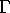be the running time of the fastest sequential algorithm. Then: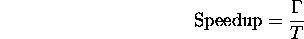What is linear speedup?

Say you have P processors. Speedup is at most P. Why? (Hint: a parallel algorithm that runs in T steps on P processors can be simulated on a sequential machine in how many steps?)

4. Work: W = TP.

Measures algorithm inefficiencies due to idle processors.

5. Efficiency of processor utilization: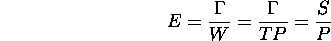6. Is it always possible to minimize T and E?

Example: consider two algorithms for solving a problem of size M:

1. M steps on an M processor machine.

2.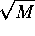steps on an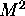processor machine.

If we have anprocessor machine and need to run the algorithm X times, which algorithm should we use? Assume theprocessor machine may be split in M M processor machines.

(Recall: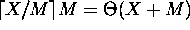.)

# Simulating a Large Array of Processors with a Small Array of Processors

Let's say we want to solve a problem of size N with P processors.

1. We generally assume that P = N. Is this valid? --- More later.

2. Suppose, we have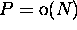fine-grain processors in a linear array. Can we sort N numbers?

Fine-grain vs. coarse-grain processing.

3. Assuming appropriate granularity, here's how we simulate 12 processors on 3 processors: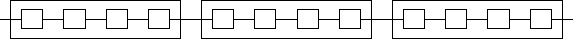What about simulating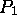processors on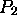processors, where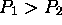?

What slowdown do we encounter?

4. Is the scaled-down algorithm less efficient?

5. So, why do we assume, in general, that P = N?

Implications:

1. Given an algorithm efficient for large P, we can construct one efficient for small P.

# Problems

1.2
Consider two algorithms for solving a a problem of size M, one that runs in M steps on an M-processor machine and one that runs insteps on an-processor machine. Which algorithm is more efficient?

Assume that the cost of running a P-processor machine for T steps is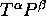, where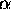and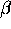are constants and P = M or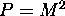. For what values ofandis the first algorithm cheaper to run? For what values ofandis the second algorithm cheaper to run?

1.3
Consider two algorithms for solving a problem of size M, one that runs in M steps on an M-processor machine and one that runs insteps on an-processor machine. Which algorithm will run faster on an N-processor machine? (Hint: Your answer depends on the relative sizes of M and N, and you will need to use the fact that an N-processor machine can simulate a P-processor machine with slowdown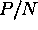.)

Thomas P. Kelliher
Fri Jan 29 10:40:33 EST 1999
Tom Kelliher